Next: 5.3 Field Calculation over Up: 5.2 Field Calculation over Previous: 5.2.3 Transfer Matrix Method

## 5.2.4 Beam Propagation Method

A totally different method was incorporated by Andreas Erdmann into the lithography simulator SOLID [144,146,147]. As this approach is based on the finite-difference beam propagation method  it is not restricted to laterally homogeneous resists. However, it is only suited for a planar topography. The field calculation is based on a numerical solution of the Helmholtz equation (cf. (4.2)) that writes inside the inhomogeneous resist as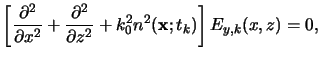(5.30)

whereby a transversal-electric polarized light and a two-dimensional simulation domain is required . The field amplitude Ey, k(x, z) is separated into a slowly varying amplitude Ak(x, z) obeying (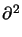/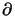z2)Ak(x, z) = 0 and an exponential factor exp(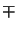jk0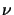z) describing the propagation upwards and downwards the resist, i.e.,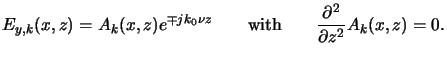(5.31)

Insertion of (5.32) into (5.31) transforms the Helmholtz equation into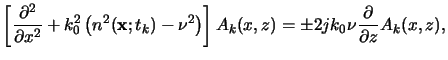(5.32)

which for example can be solved on an equidistant grid with a Crank-Nicholson scheme . The boundary conditions can either be transparent ones  used in case of isolated features, or periodic ones applied for dense lines and spaces. Consideration of reflective substrates requires additional modifications as either downward or upward propagation--but not simultaneously--can be modeled by (5.32). However, reflections occurring at the air/resist as well as the resist/substrate interface can conveniently be calculated by Fresnel's reflection formulae [11, pp. 36-51]. The light is then repeatedly propagated down and up through the resist until its intensity is negligible or, alternatively, until a fixed number of iterations is performed and then simpler methods, e.g., the transfer matrix algorithm, are employed.

In the above described form the beam propagation is only suited for low- NA applications as the beams are assumed to travel almost parallel to the vertical axis. An extended wide angle algorithm exists  that is suited for higher numerical aperture lithography simulation. Numerical problems such as poor convergence and instability of the solution occur in case of a strongly varying refractive index. A more rigorous finite-difference time domain propagation method is then required . The main limitation of this method is its restriction to planar layers. In the next section we describe various methods suited for nonplanar topography.Next: 5.3 Field Calculation over Up: 5.2 Field Calculation over Previous: 5.2.3 Transfer Matrix Method
Heinrich Kirchauer, Institute for Microelectronics, TU Vienna
1998-04-17